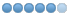# Can someone help me with this? Brain broken :)

• zusuk
•Topic Author
• Offline
• Elite Member
•• LuminariMUD Developer
10 months 1 week ago #10107 by zusuk
Someone bored and able to help me straighten this out?  I was trying to set up an exchange system for currencies and walked away from it... came back and was confused by what I had started :P
```/* exchange code */
#define ACCEXP_EXCHANGE_RATE 1500.0
#define QP_EXCHANGE_RATE 1200.0
#define GOLD_EXCHANGE_RATE 5.0
#define EXP_EXCHANGE_RATE 1.0
#define SRC_DST_ACCEXP 1
#define SRC_DST_QP 2
#define SRC_DST_GOLD 3
#define SRC_DST_EXP 4
#define NUM_EXCHANGE_TYPES 5 // one more than the last above
const char *exchange_types[NUM_EXCHANGE_TYPES] =
{
"",
"account experience",
"quest points",
"gold coins",
"experience"};
void show_exchange_rates(struct char_data *ch)
{
send_to_char(ch, "Usage: exchange <currency source> <currency purchasing> <amount to purchase>\r\n");
send_to_char(ch, "       This command is used to exchange in-game currencies including your "
"experience points, gold coins, account experience or quest points.\r\n");
send_to_char(ch, "       currencies: accexp | qp | gold | exp\r\n");
send_to_char(ch, "       current exchange rates: accexp: %d, qp: %d, gold: %d, exp: %d",
(int)ACCEXP_EXCHANGE_RATE,
(int)QP_EXCHANGE_RATE,
(int)GOLD_EXCHANGE_RATE,
(int)EXP_EXCHANGE_RATE);
return;
}
// ACMD_DECL(do_cexchange)
ACMD(do_cexchange)
{
char arg1[MAX_STRING_LENGTH] = {'\0'};
char arg2[MAX_STRING_LENGTH] = {'\0'};
char arg3[MAX_STRING_LENGTH] = {'\0'};
float amount = 0.0, cost = 0.0, pool = 0.0;
int source = 0, exchange = 0;
/*temp*/
if (GET_LEVEL(ch) < LVL_STAFF)
{
send_to_char(ch, "Under construction!\r\n");
return;
}
/*TEMP*/
three_arguments(argument, arg1, sizeof(arg1), arg2, sizeof(arg2), arg3, sizeof(arg3));
if (!*arg1 || !*arg2 || !*arg3)
{
show_exchange_rates(ch);
return;
}
/* valid arguments */
if (!is_number(arg3))
{
send_to_char(ch, "The third argument is invalid, it needs to be a number.\r\n");
show_exchange_rates(ch);
return;
}
amount = atoi(arg3);
if (amount <= 0)
{
send_to_char(ch, "The third argument needs to be above 0.\r\n");
show_exchange_rates(ch);
return;
}
if (is_abbrev(arg1, "accexp"))
source = SRC_DST_ACCEXP;
else if (is_abbrev(arg1, "qp"))
source = SRC_DST_QP;
else if (is_abbrev(arg1, "gold"))
source = SRC_DST_GOLD;
else if (is_abbrev(arg1, "exp"))
source = SRC_DST_EXP;
else
{
send_to_char(ch, "The first argument is invalid.\r\n");
show_exchange_rates(ch);
return;
}
if (is_abbrev(arg2, "accexp"))
exchange = SRC_DST_ACCEXP;
else if (is_abbrev(arg2, "qp"))
exchange = SRC_DST_QP;
else if (is_abbrev(arg2, "gold"))
exchange = SRC_DST_GOLD;
else if (is_abbrev(arg2, "exp"))
exchange = SRC_DST_EXP;
else
{
send_to_char(ch, "The second argument is invalid.\r\n");
show_exchange_rates(ch);
return;
}
if (source == exchange)
{
send_to_char(ch, "Your source and desired currency types cannot be the same.\r\n");
show_exchange_rates(ch);
return;
}
/* everything should be valid by now, so time for some math */
/* how much is this transaction going to "cost" */
switch (exchange)
{
case SRC_DST_ACCEXP:
cost = (float)ACCEXP_EXCHANGE_RATE * amount;
/* cap for account xp currently */
if ((amount + (float)GET_ACCEXP_DESC(ch)) > 33999.0)
{
send_to_char(ch, "Account experience caps at 34K.\r\n");
return;
}
break;
case SRC_DST_QP:
cost = (float)QP_EXCHANGE_RATE * amount;
break;
case SRC_DST_GOLD:
cost = (float)GOLD_EXCHANGE_RATE * amount;
break;
case SRC_DST_EXP:
cost = (float)EXP_EXCHANGE_RATE * amount;
break;
default: /* should never get here */
show_exchange_rates(ch);
send_to_char(ch, "Please report to staff: reached default case in 1st exchange switch in do_cexchange.\r\n");
return;
}
/* can we afford it? if so, go ahead and charge 'em */
switch (source)
{
case SRC_DST_ACCEXP:
pool = cost / ((float)ACCEXP_EXCHANGE_RATE); /* amount we need */
if (pool < 1.0)
{
send_to_char(ch, "You need to increase the amount for this exchange.\r\n");
return;
}
if (GET_ACCEXP_DESC(ch) < pool)
{
send_to_char(ch, "You do not have enough account exp, you need %d total.\r\n", (int)pool);
return;
}
/* bingo! */
GET_ACCEXP_DESC(ch) -= pool;
save_account(ch->desc->account);
send_to_char(ch, "You exchange %d account exp for ", (int)pool);
break;
case SRC_DST_QP:
pool = cost / ((float)QP_EXCHANGE_RATE); /* amount we need */
if (pool < 1.0)
{
send_to_char(ch, "You need to increase the amount for this exchange.\r\n");
return;
}
if (GET_QUESTPOINTS(ch) < pool)
{
send_to_char(ch, "You do not have enough quest points, you need %d total.\r\n", (int)pool);
return;
}
/* bingo! */
GET_QUESTPOINTS(ch) -= pool;
send_to_char(ch, "You exchange %d quest points for ", (int)pool);
break;
case SRC_DST_GOLD:
pool = cost / ((float)GOLD_EXCHANGE_RATE); /* amount we need */
if (pool < 1.0)
{
send_to_char(ch, "You need to increase the amount for this exchange.\r\n");
return;
}
if (GET_GOLD(ch) < pool)
{
send_to_char(ch, "You do not have enough gold on hand, you need %d total on "
"hand (not in bank) to make the exchange.\r\n",
(int)pool);
return;
}
/* bingo! */
GET_GOLD(ch) -= pool;
send_to_char(ch, "You exchange %d gold for ", (int)pool);
break;
case SRC_DST_EXP:
pool = cost / ((float)EXP_EXCHANGE_RATE); /* amount we need */
if (pool <= 0.0)
{
send_to_char(ch, "You need to increase the amount for this exchange.\r\n");
return;
}
if (GET_EXP(ch) < pool)
{
send_to_char(ch, "You do not have enough experience points, you need %d total.\r\n", (int)pool);
return;
}
/* bingo! */
GET_EXP(ch) -= pool;
send_to_char(ch, "You exchange %d experience points for ", (int)pool);
break;
default: /*shouldn't get here*/
show_exchange_rates(ch);
send_to_char(ch, "Please report to staff: reached default case in source switch in do_exchange.\r\n");
return;
}
/* final portion of transaction, give them their purchase */
switch (exchange)
{
case SRC_DST_ACCEXP:
send_to_char(ch, "%d account experience.", (int)amount);
GET_ACCEXP_DESC(ch) += (int)amount;
save_account(ch->desc->account);
break;
case SRC_DST_QP:
send_to_char(ch, "%d quest points.", (int)amount);
GET_QUESTPOINTS(ch) += (int)amount;
break;
case SRC_DST_GOLD:
send_to_char(ch, "%d gold coins.", (int)amount);
GET_GOLD(ch) += (int)amount;
break;
case SRC_DST_EXP:
send_to_char(ch, "%d experience points.", (int)amount);
GET_EXP(ch) += (int)amount;
break;
default: /* should never get here */
show_exchange_rates(ch);
send_to_char(ch, "Please report to staff: reached default case in 2nd exchange switch in do_cexchange.\r\n");
return;
}
return;
}
#undef ACCEXP_EXCHANGE_RATE
#undef QP_EXCHANGE_RATE
#undef GOLD_EXCHANGE_RATE
#undef EXP_EXCHANGE_RATE
#undef SRC_DST_ACCEXP
#undef SRC_DST_QP
#undef SRC_DST_GOLD
#undef SRC_DST_EXP
/*end exchange code */
```

Website
www.luminariMUD.com

Main Game Port
luminariMUD.com:4100